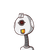# ii.In figure, points X, Y, Z are the midpoints of side AB side BC and side AC ofangle ABC respectively. AB = 5cm , AC

ii.
In figure, points X, Y, Z are the midpoints of side AB side BC and side AC of
angle ABC respectively. AB = 5cm , AC= 9cm and BC = 11 cm. Find the length
of XY, YZ, XZ.​

### 1 thought on “ii.<br />In figure, points X, Y, Z are the midpoints of side AB side BC and side AC of<br />angle ABC respectively. AB = 5cm , AC”

1.Given that X,Y and Z are the midpoints of the side AB,BC and CA respectively.

By midpoint theorem

XZ∣∣BC and XZ =

2

1

BC

BC=11cm

⇒XZ=

2

BC

=

2

11

=5.5cm

Similarly,

XY∣∣AC and XY =

2

1

AC

AC=9cm

XY=

2

AC

=

2

9

=4.5 cm

YZ∣∣AB and YZ =

2

1

AB

AB=5cm

YZ=

2

AB

=

2

5

=2.5 cm